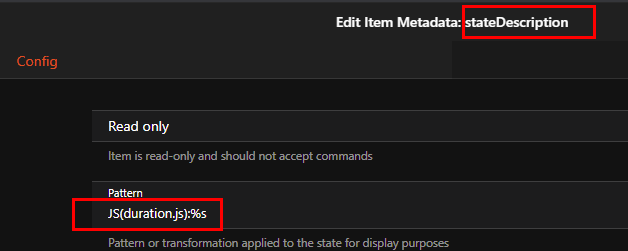OH3: How to create item labels with state presentation?

Recently I was playing around with openHAB3 (RC1) and tried different item migration possibilities:

1. creation of items directly in MainUI
2. copy&paste from existing textual OH2.x config (expert mode)
3. textual creation in OH3

Some of my old items in the OH2.x config contains state presentation such as:
Number zwayPlug2Energy "Verbrauch [%.1f kWh]" ...

But so far I was not able to create such items with No.1 and No.2. With the textual creation it is working but I would like to use MainUI only in the future.

Could someone give me a hint what I can try?

2 Likes

Wow, that was easy! I guess, I missed this in the tutorials.

Thank you!!!

Hello I have a question on the subject,

can one at the place a numerical value also directly divide or convert. For example, a time in seconds convert into hours.

Thanks!

Without scripting it’s not possible. But the script itself is quite easy.

// computes nicely formatted duration from given minutes
(function(i){

var d = Math.floor(i / (24 * 60));
var h = Math.floor((i / 60) - (24 * d));
var m = Math.round(i - 60 * (24 * d + h));
var result = '';

// days
if (d > 0) {
if(d == 1)
result = result + d + ' Day';
else
result = result + d + ' Days ';
}

if(h > 0)
{
if(h == 1)
result = result + ("00" + h).substr(-2,2) + " Hour "
else
result = result + ("00" + h).substr(-2,2) + " Hours "
}

result = result + ("00" + m).substr(-2,2) + " Minutes";

return result;
})(input)

Save that e.g. as duration.js within the scripts folder and add it within the state description metadata of the item that reports seconds.But the script converts into days/hours/minutes/seconds and not only hours

Hello,

thank you for the quick reply! Have the script slightly adjusted since the input variable is seconds with me. Functioning now super!

Greetings

// computes nicely formatted duration from given seconds
(function(i){

var d = Math.floor(i / (24 * 60 * 60));
var h = Math.floor((i / (60 * 60)) - (24 * d));
var m = Math.round(i / 60 -(60 * (24 * d + h)));
var s = Math.round(i - 60 * (m + (60 * (24 * d + h))));

var result = '';

// days
if (d > 0) {
if(d == 1)
result = result + d + ' Tag';
else
result = result + d + ' Tage ';
}

if(h > 0)
{
if(h == 1)
result = result + ("00" + h).substr(-2,2) + " Stunde "
else
result = result + ("00" + h).substr(-2,2) + " Stunden "
}

result = result + ("00" + m).substr(-2,2) + " Minuten ";
// result = result + ("00" + s).substr(-2,2) + " Sekunden";
return result;
})(input)